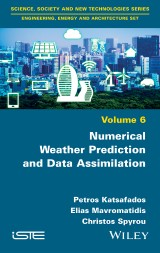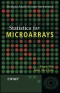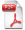# Details## Numerical Weather Prediction and Data Assimilation

1. Aufl.
 139,99 € Verlag: Wiley Format: EPUB Veröffentl.: 16.07.2020 ISBN/EAN: 9781119701019 Sprache: englisch Anzahl Seiten: 224

DRM-geschütztes eBook, Sie benötigen z.B. Adobe Digital Editions und eine Adobe ID zum Lesen.

## Beschreibungen

#### Titelbeschreibung

This book has as main aim to be an introductory textbook of applied knowledge in Numerical Weather Prediction (NWP), which is a method of weather forecasting that employs: A set of equations that describe the flow of fluids translated into computer code, combined with parameterizations of other processes, applied on a specific domain and integrated in the basis of initial and domain boundary conditions. Current weather observations serve as input to the numerical computer models through a process called data assimilation to produce atmospheric properties in the future (e.g. temperature, precipitation, and a lot of other meteorological parameters). Various case studies will be also presented and analyzed through this book.

#### Inhaltsverzeichnis

<p>Preface vii</p> <p>Introduction ix</p> <p><b>Chapter 1. The Primitive Equations </b><b>1</b></p> <p>1.1. Wind forecast equations (conservation of momentum) 1</p> <p>1.1.1. Real forces 6</p> <p>1.1.2. Apparent forces 14</p> <p>1.1.3. Equation of motion 17</p> <p>1.2. Continuity equation (conservation of mass) 19</p> <p>1.3. Temperature forecast equation (conservation of energy) 21</p> <p>1.4. Moisture forecast equation (conservation of water vapor) 26</p> <p>1.5. Synopsis of equations 26</p> <p><b>Chapter 2. Solving Methods in NWP Models </b><b>31</b></p> <p>2.1. Decomposition of variables – the perturbation method 31</p> <p>2.1.1. Synoptic scale equations 39</p> <p>2.1.2. Mesoscale equations 40</p> <p>2.2. Numerical solutions of partial differential equations 43</p> <p>2.2.1. Computations by finite difference schemes 44</p> <p>2.2.2. Derivation of finite difference representations 46</p> <p>2.2.3. Methods for solving the advection–diffusion equation 50</p> <p>2.3. Time splitting 63</p> <p><b>Chapter 3. Domain Structures and Boundary Conditions </b><b>67</b></p> <p>3.1. Horizontal grid structure and resolution 68</p> <p>3.2. The vertical coordinate system 71</p> <p>3.2.1. Terrain-following coordinate system 72</p> <p>3.3. Boundary conditions 86</p> <p>3.3.1. Lateral boundary conditions 88</p> <p>3.3.2. Upper (top) boundary conditions 90</p> <p>3.3.3. Lower (bottom) boundary conditions 90</p> <p>3.4. Design of a simulation 99</p> <p><b>Chapter 4. Introduction to Data Assimilation </b><b>101</b></p> <p>4.1. Successive correction methods 102</p> <p>4.2. Least square method 103</p> <p>4.3. Variational approach 108</p> <p>4.4. Generalization of the methods 114</p> <p><b>Chapter 5. Desert Dust Modeling </b><b>119</b></p> <p>5.1. Dust uptake mechanisms formulation 121</p> <p>5.2. Dust advection and deposition 127</p> <p>5.3. Parameterization of the dust feedbacks on climate 132</p> <p><b>Chapter 6. Simulations of Extreme Weather and Dust Events </b><b>143</b></p> <p>6.1. Case study 1: numerical simulation of a Mediterranean cyclone and its sensitivity on lower boundary conditions 143</p> <p>6.1.1. Description of the synoptic conditions 143</p> <p>6.1.2. Design of the simulations 145</p> <p>6.1.3. Analysis of the numerical simulations 147</p> <p>6.2. Case study 2: nowcasting an extreme precipitation event 151</p> <p>6.2.1. Synoptic analysis of the event 151</p> <p>6.2.2. Nowcasting methodology and results 153</p> <p>6.3. Case study 3: seasonal predictability of a large-scale heat wave 157</p> <p>6.3.1. Description of the synoptic conditions 158</p> <p>6.3.2. Model description and methodology 159</p> <p>6.3.3. Predictability assessment 161</p> <p>6.4. Numerical study of a severe desert dust storm over Crete 163</p> <p><b>Appendices </b><b>169</b></p> <p>Appendix 1 171</p> <p>Appendix 2 179</p> <p>References 197</p> <p>Index 207</p>

#### Autorenportrait

<p><b>Petros Katsafados</b>, Associate Professor, Department of Geography, Harokopio University, Athens, Greece.</p> <p><b>Elias Mavromatidis</b>, Research Associate, Department of Geography, Harokopio University, Athens, Greece.</p> <p><b>Christos Spyrou</b>, Researcher, National and Kapodistrian University of Athens, Athens, Greece.</p>

US

## Diese Produkte könnten Sie auch interessieren:Statistics for Microarrays
von: Ernst Wit, John McClure90,99 €